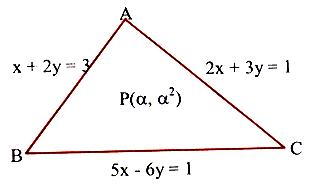×#### Thank you for registering.

One of our academic counsellors will contact you within 1 working day.

Click to Chat

1800-1023-196

+91-120-4616500

CART 0

• 0

MY CART (5)

Use Coupon: CART20 and get 20% off on all online Study Material

ITEM
DETAILS
MRP
DISCOUNT
FINAL PRICE
Total Price: Rs.

There are no items in this cart.
Continue Shopping```Position of two points with respect to a given lineLet the line be ax + by + c = 0 and P(x1, y1), Q(x2, y2) be two points.

Case 1:

If P(x1, y1) and Q(x2, y2) are on the opposite sides of the line
ax + by + c = 0, then the point R on the line ax + by + c = 0 divides the line PQ internally in the ratio m1 : m2, where m1/m2 must be positive.

Co-ordinates of R

are (m1x2+m2x1/m1+m2, m1y2+m2y1/m1+m2).

Point R lies on the line ax + by + c = 0.

⇒ m1/m2 = ax1+by1+c/ax2+by2+c > 0So that ax1 + by1 + c and ax2 + by2 + c should have opposite signs.

Case 2:

If ax1 + by1 + c and ax2 + by2 + c have the same signs then m1/m2 = –ve, so that the point R on the line ax + by + c = 0 will divide the line PQ externally in the ratio m1 : m2 and the points P(x1, y1) and Q(x2, y2) are on the same side of the line ax + by + c = 0.

Illustration:

Find the range of θ in the interval (0, π) such that the points (3, 5) and (sinθ, cosθ) lie on the same side of the line x + y – 1 = 0.

Solution:

3 + 5 – 1 =7 > 0 ⇒ sinθ + cosθ – 1 > 0

⇒ sin(π/4 + θ) > 1/√2

⇒ π/4 < π/4 + θ < 3π/4

⇒ 0 < θ < π/2.

Illustration:

Find a, if (α, α2) lies inside the triangle having sides along the lines

2x + 3y = 1, x + 2y – 3 = 0, 6y = 5x – 1.

Solution:

Let A, B, C be vertices of the triangle.

A ≡ (–7, 5), B ≡ (5/4, 7/8),

C ≡ (1/3, 1/9).

Sign of A w.r.t. BC is –ve.If p lies in-side the ¦ABC, then sign of P will be the same as sign of a w.r.t. the line BC

⇒     5α – 6α2 – 1 < 0.                         …… (1)

Similarly    2α + 3α2 – 1 > 0.                                …… (2)

And,          α + 2α2 – 3 < 0.                          …… (3)

Solving, (1), (2) and (3) for α and then taking intersection,

We get      α ? (1/2, 1) ∪ (–3/2, –1).

Illustration:

The equations of the perpendicular bisectors of the sides AB and AC of a triangle ABC are respectively x – y + 5 = 0 and x + 2y = 0. If the co-ordinates of A are (1, –2), find the equation of BC.

Solution:

From the figure,

E ≡ (x1+1/2, y1–2/2),

F ≡ (x2+1/2, y2–2/2).Alt text : Equations of the perpendicular bisectors of sides of triangle

Since E and F lie on OE and OF respectively,

x1 – y1 + 13 = 0                                  … (1)

and   x2 + 2y2 – 3 = 0                                  … (2)

Also, slope of AB = –1 and slope of AC is 2, so that

x1 + y1 + 1 = 0.                                  … (3)

And   2x2 – y2 – 4 = 0                                  … (4)

Solving these equations, we get the co-ordinates of B and C as

B ≡ (–7, 6) and C ≡ (11/5, 2/5)

⇒ Equation of BC is 14x + 23y – 40 = 0.

Illustration:

Two fixed points A and B are taken on the co-ordinate axes such that OA = a and OB = b. Two variable points A’ and B’ are taken on the same axes such that OA’ + OB’ = OA + OB. Find the locus of the point of intersection of AB’ and A’B.

Solution:

Let A ≡ (a, 0), B (0, b), A’ ≡ (a’, 0), B’ ≡ (0, b’).

Equation of A’B is x/a' + y/b' = 1.                                          …. (1)

and equation of AB’ is x/a + y/b'  = 1.                                    …. (2)

Subtracting (1) from (2), we get, x (1/a – 1/a') + y(1/b' – 1/b) = 0.

⇒ x(a'–a)/aa' + y(b–b')/bb' = 0.                             [Using a’ – a = b – b’]

⇒ x/a(b–b'+a) + y/bb', 0 ⇒ b’ = a(a+b)y/ay–bx.                              ….. (3)

From (2) b’x + ay = (4) we get x + y = a + b

which is the required locus.

To read more, Buy study materials of Straight Lines comprising study notes, revision notes, video lectures, previous year solved questions etc. Also browse for more study materials on Mathematics here.

```### Course Features

• 731 Video Lectures
• Revision Notes
• Previous Year Papers
• Mind Map
• Study Planner
• NCERT Solutions
• Discussion Forum
• Test paper with Video Solution